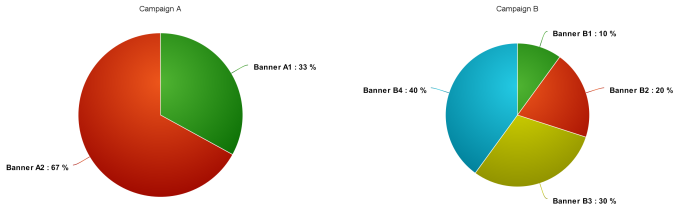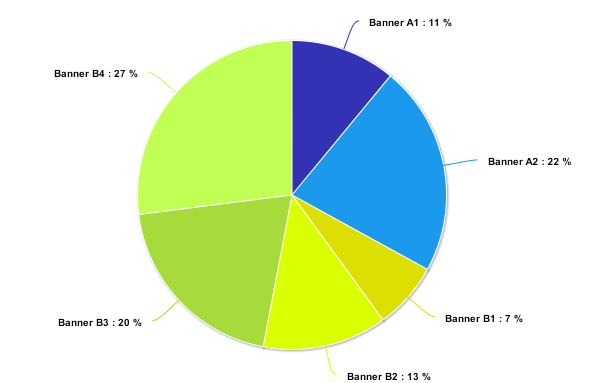# Campaigns/Banners Manual Weighting

Epom Ad Server provides a flexible mechanism for the prioritization of Campaigns and Banners

The priorotization is done either by changing the weight value of the Campaigns and/or Banners, by adding the Campaign to a certain priority group, or both.

## Setting the Campaign or Banner Weight

The Weight can be set in the corresponding fields on the Campaign and Banner cards.

📘

Note!

Banner-level weights only influence the display probability for banners within the campaign to which they belong.

## Probability Сalculation

Let us assume that we have two campaigns (Campaign A and Campaign B). There are two banners (Banner A1 and Banner A2) in Campaign A and four banners (Banner B1, Banner B2, Banner B3 and Banner B4) in Campaign B.

Weights for banners:

Banner A1 = 1; Banner A2 = 2;

Banner B1 = 1; Banner B2 = 2; Banner B3 = 3; Banner B4 = 4;

Banner probability to be shown within the campaign is calculated according to the following formula:

Banner # Probability = Banner # Weight/(Banner #1 Weight + Banner #2 Weight + ... + Banner N Weight)

So, for our example the weights will be:

Campaign A

Banner A1 Probability = Banner A1 Weight/(Banner A1 Weight + Banner A2 Weight) = 1/(1+2) = 1/3 = ~ 33,3%

Banner A2 Probability= Banner A2 Weight/(Banner A1 Weight + Banner A2 Weight) = 2/(1+2) = 2/3 = ~ 66,6%

Campaign B

Banner B1 Probability = Banner B1 Weight/(Banner B1 Weight + Banner B2 Weight + Banner B3 Weight + Banner B4 Weight) = 1/(1+2+3+4) = 1/10 = 10%

Banner B2 Probability = Banner B2 Weight/(Banner B1 Weight + Banner B2 Weight + Banner B3 Weight + Banner B4 Weight) = 2/(1+2+3+4) = 2/10 = 20%

Banner B3 Probability = Banner B3 Weight/(Banner B1 Weight + Banner B2 Weight + Banner B3 Weight + Banner B4 Weight) = 3/10 = 30%

Banner B4 Probability= Banner B4 Weight/(Banner B1 Weight + Banner B2 Weight + Banner B3 Weight + Banner B4 Weight) = 4/(10) = 40%Now, lets set the Weight values for the campaigns:

Campaign A = 1, Campaign B = 2;

Campaigns' probability is counted according to the similar formula:

Campaign # Probability = Campaign # Weight/(Campaign #1 Weight + Campaign #2 Weight + ... + Campaign N Weight)

Campaign A Probability = Campaign A Weight/(Campaign A Weight + Campaign B Weight) = 1/(1+2) = 1/3 ~ 33,3%

Campaign B Probability = Campaign B Weight/(Campaign A Weight + Campaign B Weight) = 2/(1+2) = 2/3 ~ 66,6%

If you link both the Campaigns to one placement, the Weights of Banners will be adjusted according to the Campaigns' Weights:

Banner # Probability = Banner # Probability * Campaign # Probability

Campaign A

Banner A1 Probability = Banner A1 Probability * Campaign A Probability = (1/3) * (1/3) = 1/9 ~ 11%

Banner A2 Probability = Banner A2 Probability * Campaign A Probability = (2/3) * (1/3) = 2/9 ~ 22%

Campaign B

Banner B1 Probability = Banner B1 Probability * Campaign B Probability = (1/10) * (2/3) = 2/30 = 1/15 ~ 7%

Banner B2 Probability = Banner B2 Probability * Campaign B Probability = (2/10) * (2/3) = 4/30 = 2/15 ~ 13%

Banner B3 Probability = Banner B3 Probability * Campaign B Probability = (3/10) * (2/3) = 6/30 = 1/5 = 20%

Banner B4 Probability = Banner B4 Probability * Campaign B Probability = (4/10) * (2/3) = 8/30 = 4/15 ~ 27%## Setting Campaign Priority Group

You can use Priority Groups as an alternative to Campaign and Banner Weights.

This feature will allow you to set predefined Priority levels to your Campaigns, which will identify the order in which these ads will be requested. You may choose one of the following Priority Groups:

• Standard - default, second priority.
• Non-guaranteed - lower level, third priority.
• House ads - last priority, ads are only delivered if no other ads are available. House Ad Campaign allows you to fill unsold inventory or promote your own services. If House Ad priority is set for the Campaign, its weight is set to zero, but the weights on the Banner level work as usual.

Ads from the highest Priority Group will always be displayed if they are available.

📘

Note!

Ads from the same Priority Group will be shown according to their Weights.

Let's assume that the Banners from these two Campaigns are linked to the same Placement:

#### Campaign A

• Banner A1 with Weight: 100
• Banner A2 with Weight: 50

#### Campaign B

Priority - Non-guaranteed.

• Banner B1 with Weight: 500
• Banner B2 with Weight: 1000
• Banner B3 with Weight: 1500

In this case the ads will be displayed in the following way:

1. Campaign A Banners will always be displayed as their Campaign has the Premium priority. These Banners will be shown to users according to their Weights:
• Banner A1: ~67%
• Banner A2: ~33%
2. Only when the Campaign A Banners can not be served due to Capping, Limits, or do not meet the ad request, Campaign B Banners will be requested and served:
• Banner B1: ~17%
• Banner B2: ~33%
• Banner B3: ~50%